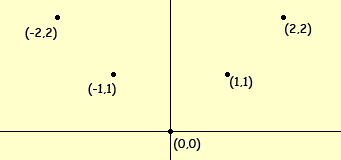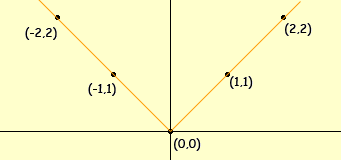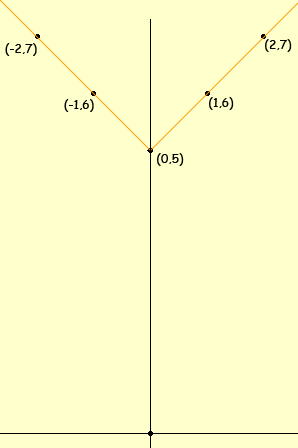Subject: vertex of an equation
Name: Pam
Who are you: Student

what is the process for finding the vertex point for an equation such as this, y= |x| - 10. Thank You

Hi Pam,

Do you know the shape of the graph of y = |x|? If not then plot some points to example the shape.

x y
0 0
1 1
2 2
-1 1
-2 2

y = |x|

Now plot the pointsand sketch the graphGraph of y = |x|

This is a useful graph to know.

Now you want to modify the function, for example to plot the graph of y = |x| + 5. The relationship between y = |x| and y = |x| + 5 is that in the second expression, for each value of x the y value is increased by 5. Thinking of the graph, the y-values are shifted upwards by 5 units. Thus the graph isgraph of y = |x| + 5

Penny